# You're calculating population density incorrectly

The question “how do you calculate population density?” sounds like the kind of thing you’d see on a third grade pop quiz. Obviously, you would calculate it in the same way you would calculate any other type of density: you take the feature of interest and its population, and divide by area.

The issue is that this approach assumes that every part of the region of interest is equally valuable.

## Why do we care about density?

For example, consider the following two islands: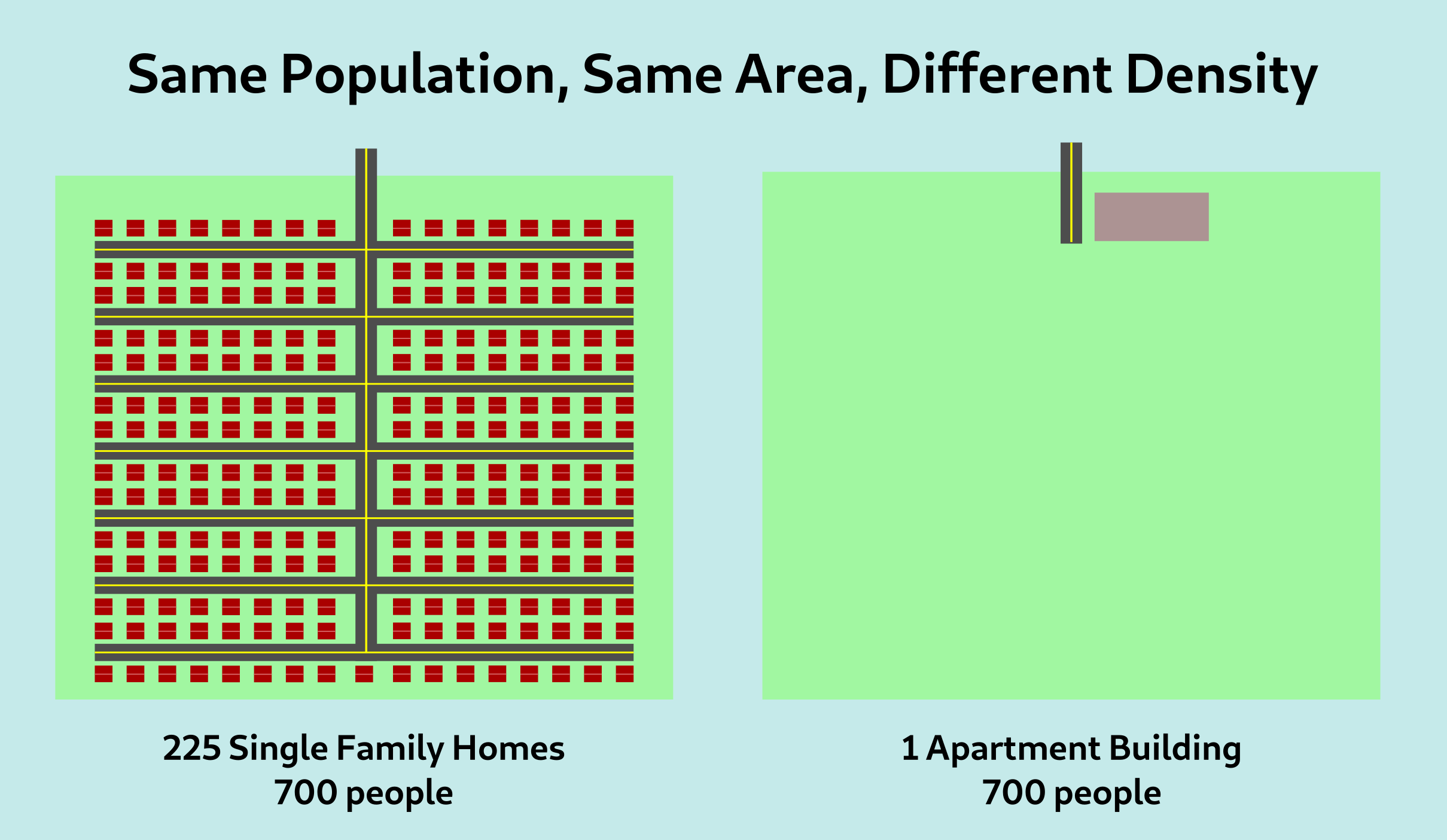In both islands, the population is 700 people, and the areas are also identical. So by a standard population density metric, the two islands are the same “density”. This metric, however, misses something. The island on the right is far more dense than the one on the left, as perceived by the typical inhabitant of the island.

In practice, when we refer to density, we’re really referring to the density as experienced by people. Walkability, the viability of public transit, social trust, access to services nearby: all of these have to do with people and their interactions with other people. As such, a density metric that does not account for this will inevitably fail to capture that relevant context.

## What is standard density measuring?

This seems like a strange question – obviously, it is measuring the mean number of people in every square meter of ground. However, another way to think about this is to divide our region up into smaller subregions; for example, let’s say each island is 1500m on a side and divide them into 9 regions each of 500m by 500m.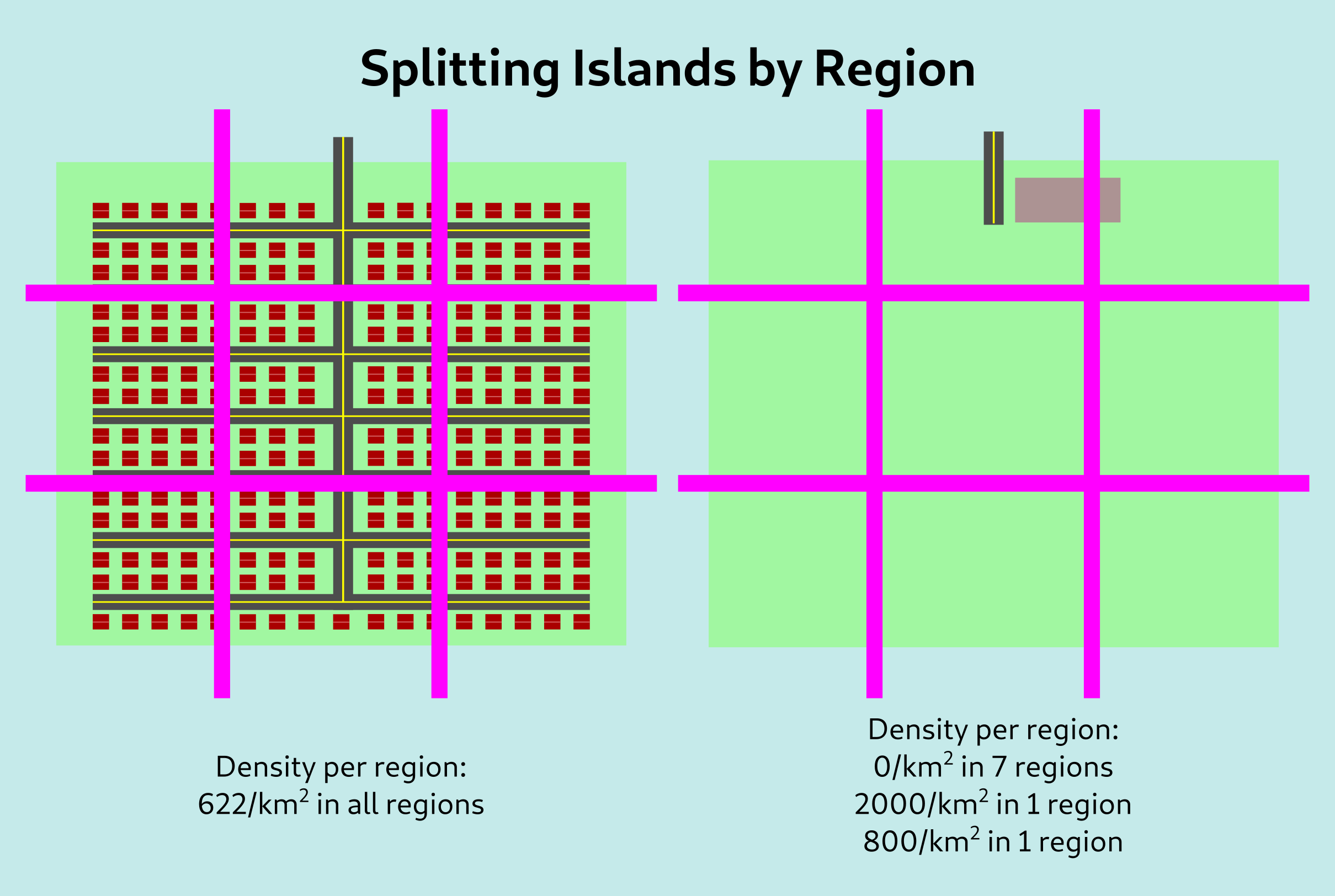To calculate normal density, we would take the average of every region, weighted by its area, to get 622/km2 in both of these cases. However, if we take the average of every region weighted by the population, we still get 622/km2 for the left island but we get 1657/km2 for the second island. This better reflects our intuition about the second island being more dense.

## A practical metric

Of course, the issue with the above approach is the grid. If we had displaced the grid slightly to the right so the entire apartment building fell within it, we would have ended up with a density of 2800/km2, and this kind of sensitivity to minor changes is really not what we want in a metric.

Instead of looking at a grid, we can instead look at disks of some radius, with one disk centered around each person. We compute the density in each disk, and then take the average of all these values across the region to compute the overall density.

## Maps

And now for some maps! Depicted here are maps of the metric for radii of 250m, 1km, and 4km, based on 2020 census data.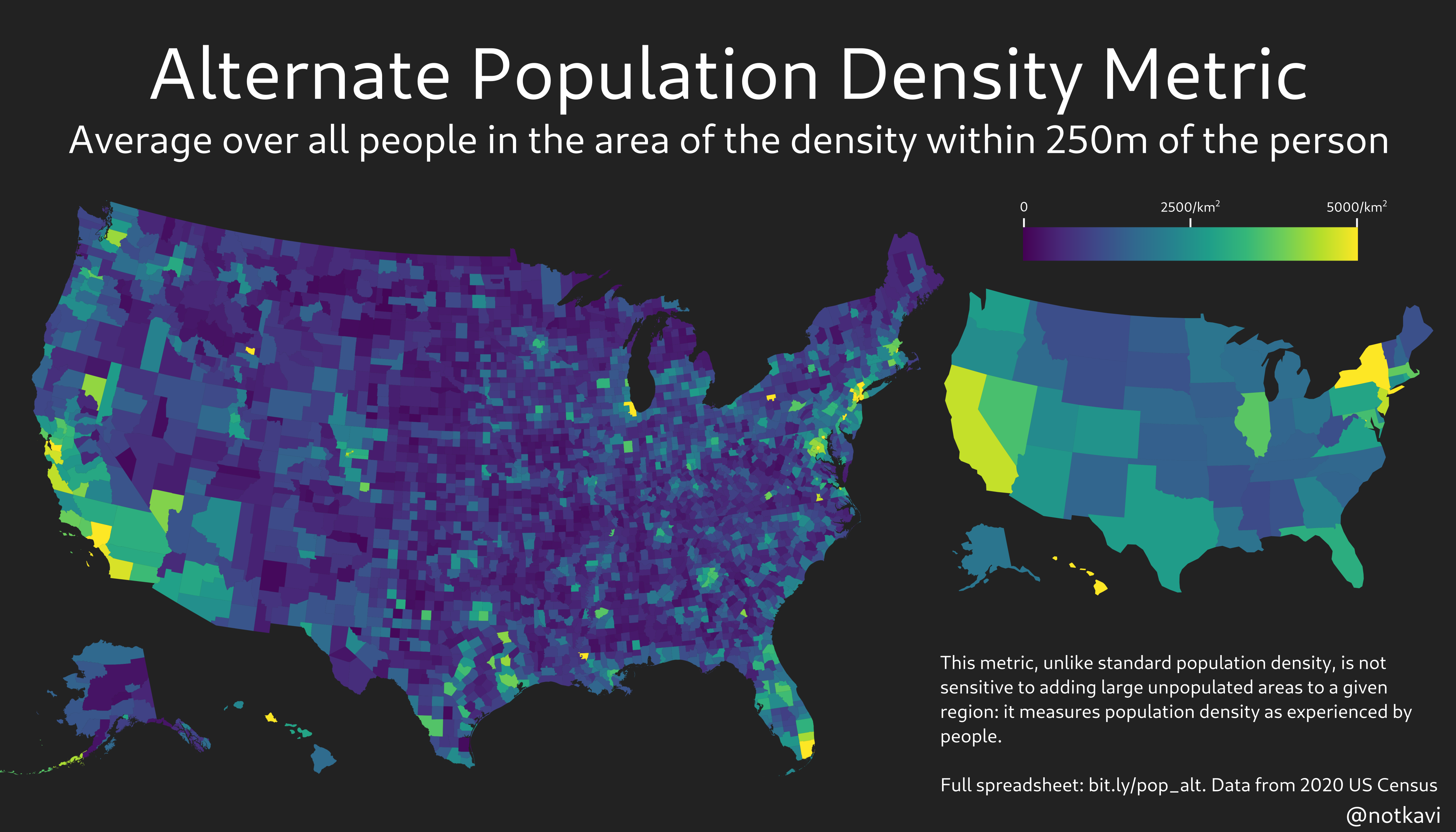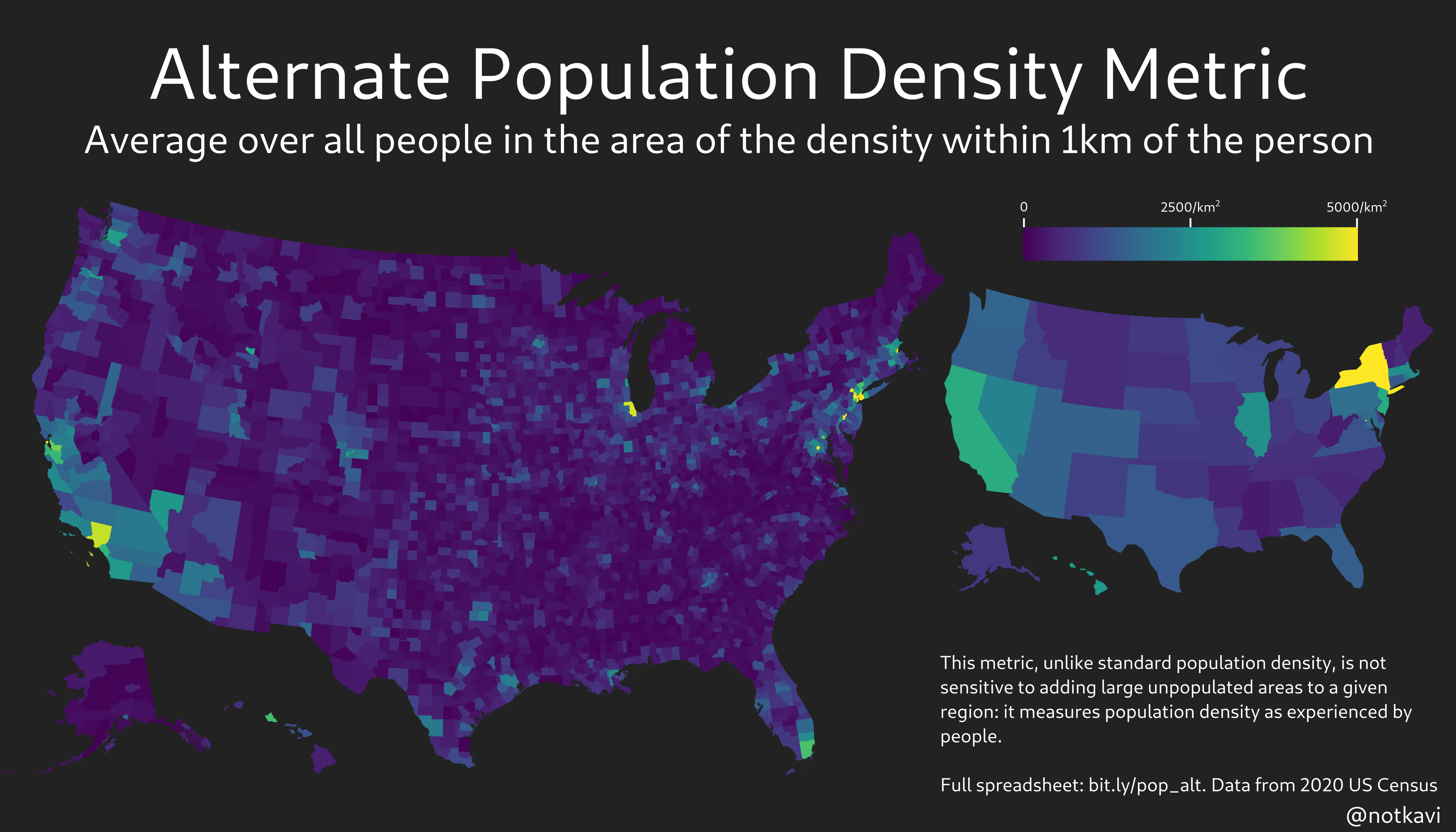Note that New York State is easily the densest state by any of these metrics, which makes sense since most people in New York State live in very urban areas!

## Political analysis

We all know that density predicts electoral outcomes right? Well, here’s what density vs Biden’s share looks like using several different metrics, by county.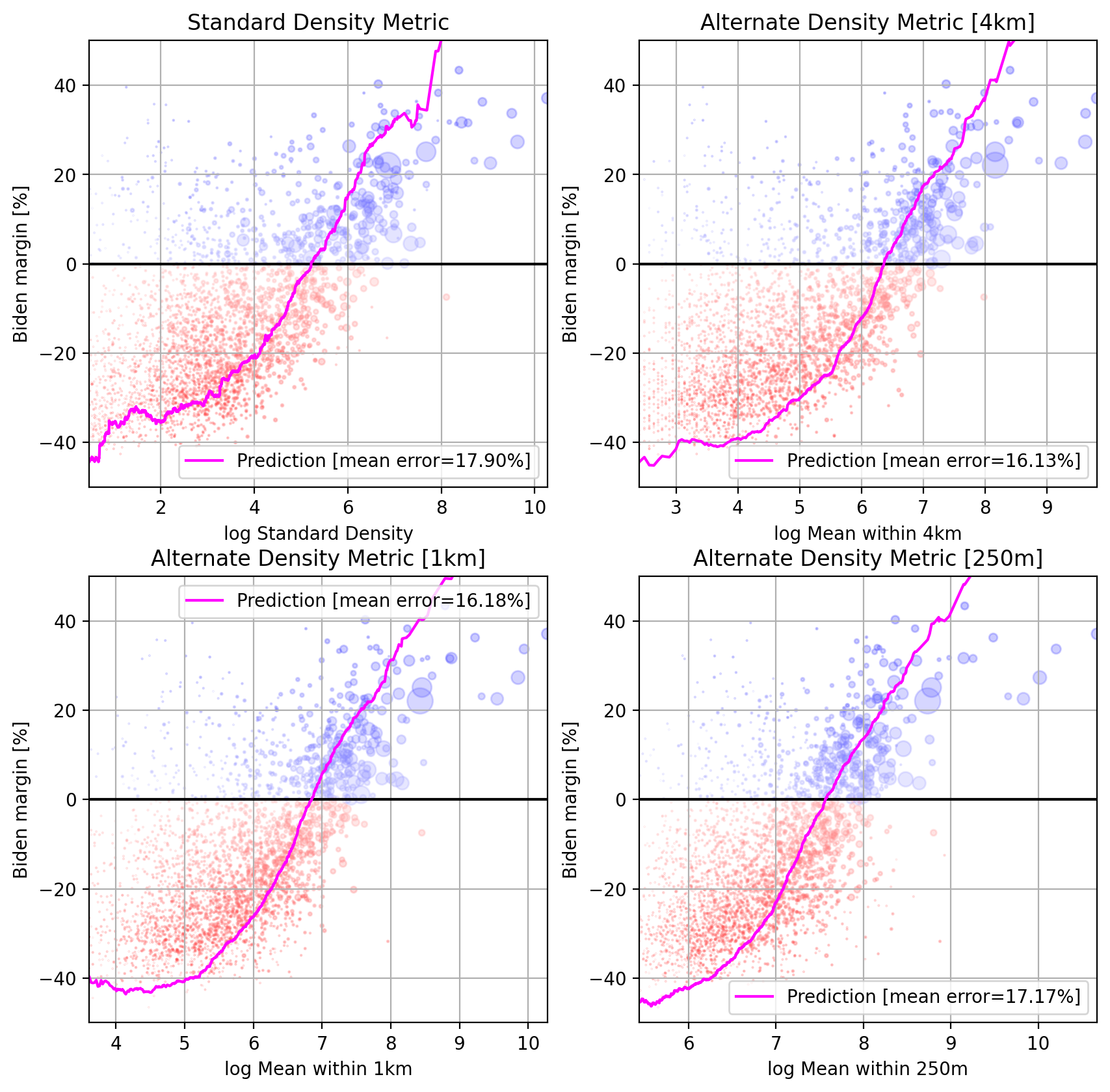Note that the mean error is lower for the alternate density metric, but by 250m, it starts rising again. This is probably due to the fact that the census data used to construct this is block level, so noise from the exact placement of the blocks starts becoming relevant below 1km or so.

We can also map out the predictions and see what’s going on: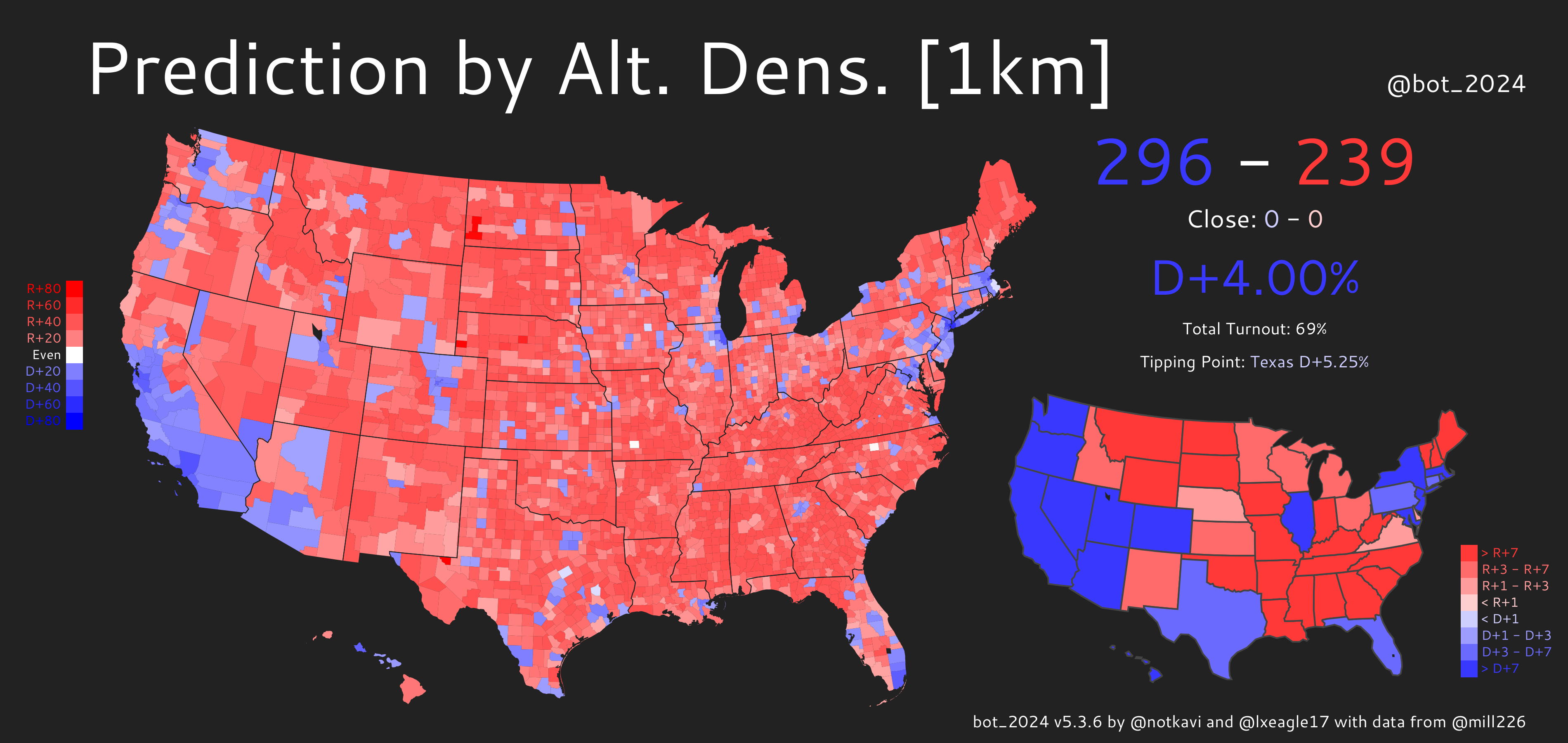You can see that there’s far fewer blue counties by either of these metrics than Biden actually received, but the alternate density metric produces a better map as it can identify large counties that are nonetheless densely populated, especially in the Western US, where counties tend to be much larger.

We can also see this on the residual maps: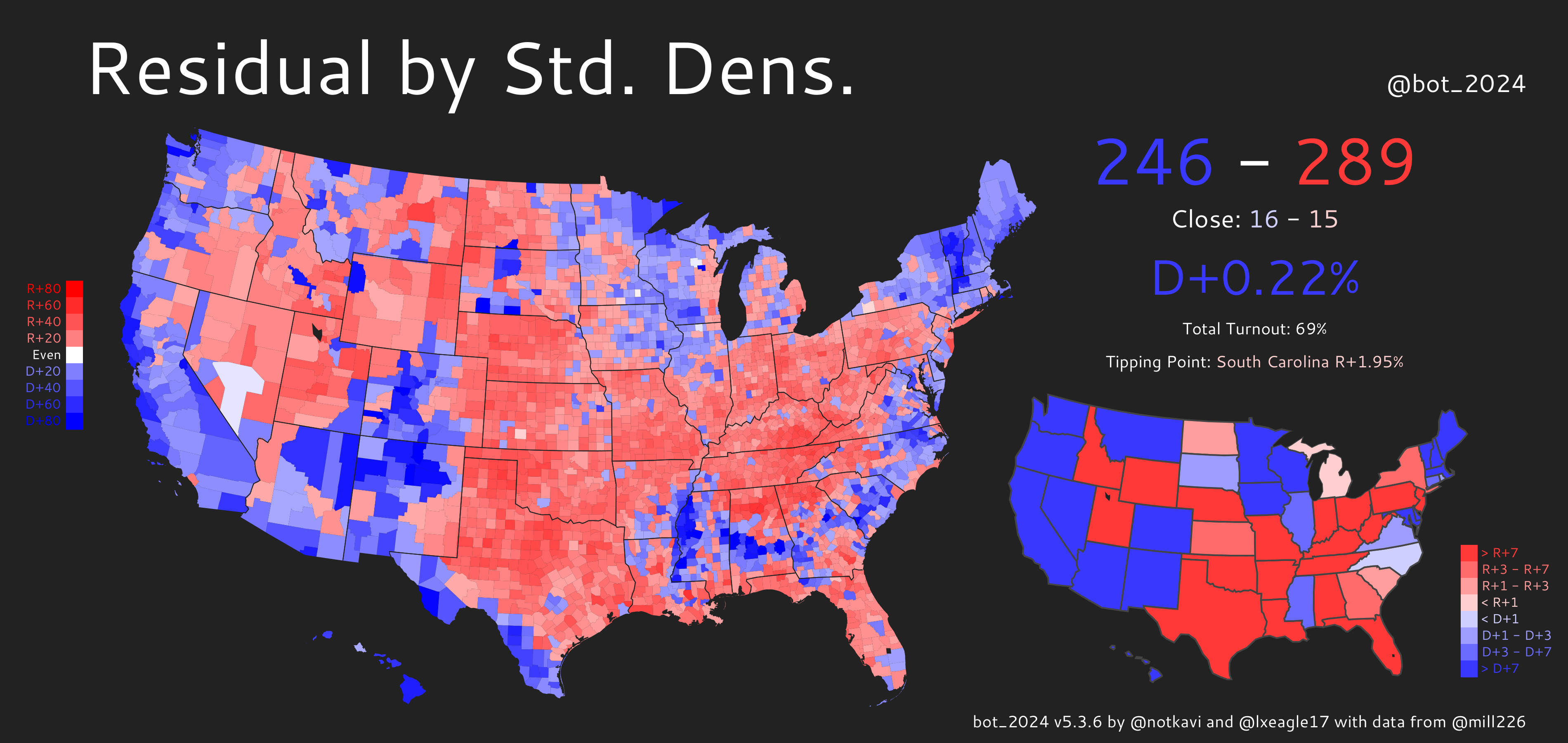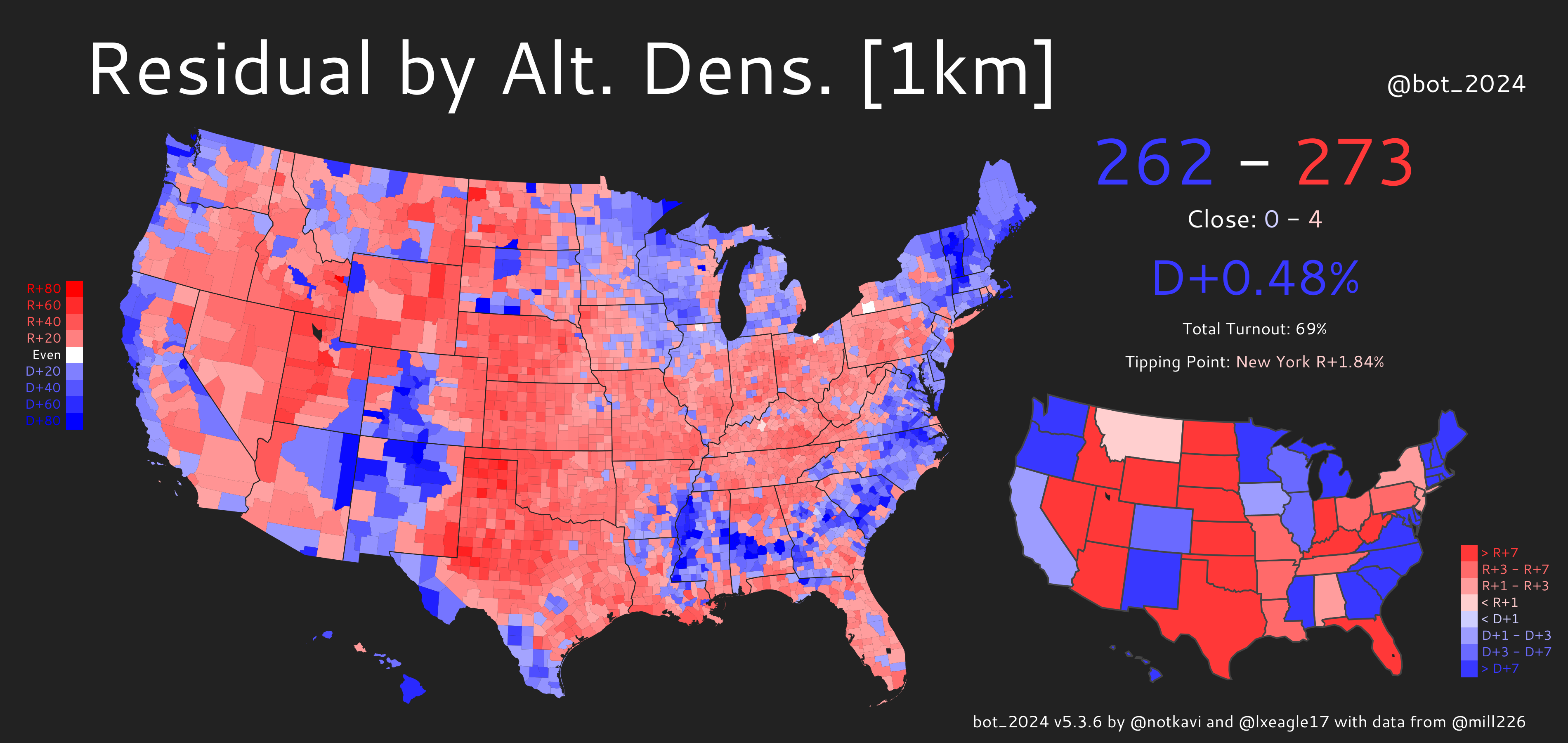Both maps show obvious patterns where the Great Plains, Appalachia, Utah, and Florida are redder than expected by density, while Native, Hispanic, Black, and secular areas, along with the midwestern Driftless area, are bluer than expected. However, you can see that standard density has an additional pattern where pretty much the entire west is much bluer than expected. This is because the west tends to have large counties such as San Bernardino and Riverside counties (California), Clark County (Nevada), or Maricopa County (Arizona) that are large in area, but are not actually nearly as sparse as the standard density metric would suggest.

One interesting sidenote: in both predictions, the tipping point state’s margin is more Democratic than the popular vote margin. This means that in a neutral, D/R+0 political environment, the Democrats would win!

Consequently, we can see that when only accounting for urban areas being more Democratic, the EC/PV gap would favor Democrats, unlike our current political reality, in which it favors Republicans. One direct implication of this is that the Electoral College actually favors urban areas over rural ones!

## Conclusion

Anyways, I hope you enjoy and have something to think about when it comes to calculating density! You can view and download the values by county here. Please let me know if you have any comments, you can reach me at @notkavi on twitter. Thanks to @lxeagle17 for helping with editing, I lack basic grammar skills.

# BAN ALL AMBIGUOUS MATHEMATICAL EXPRESSIONS

If you’re on twitter or Facebook you’ve probably seen this expression (or a variant with different numbers) before

6 ÷ 2(1+2)


The question that goes with it is “can you solve this?” and there’s usually a massive debate among people in the comments about whether the answer is 9 (corresponding to dividing first then multiplying by (1+2)) or 1 (corresponding to multiplying first to get 6 then dividing).

# Order of Operations

One thing people often seem to be arguing about is PEMDAS/BODMAS: the system of precedence used to do these operations. The acronyms suggest a sequencing of multiplication and division, but the convention taught with them is that the two operations have equal precedence and should be left associatively evaluated. So the formula would parse as (6 ÷ 2)(1+2) and thus be equal to 9.

One common response to this from mathematicians and other more sophisticated observers is that people are arguing about a completely arbitrary convention, and really you should just parenthesize better. These folks are correct, but I think they’re missing something that makes this particular example unique. For example, I think most people would correctly recall and apply the PEMDAS rule to the formula

6 - 1 + 3


I think most people would even correctly apply the rule to the formula

6 ÷ 2 × (1 + 3)


# The implicit multiplication operator

If you saw the following equation, how would you parenthesize it?

T = PV/nR


I think most people would correctly parenthesize this as T = (PV)/(nR) not T = P(V/n)R.

Most folks would say you should probably still parenthesize to be sure, but many people would casually just write PV / nR and be understood by 100% of their target audience. In a textbook you’d write $$\frac{PV}{nR}$$ and that’s how the mind reads it.

There’s even some cases where a textbook would actually use the / operator!

If you’ve taken an abstract algebra class, you’ve seen a function type written as something like

f : Z/2Z × Z/2Z -> Z


Ignoring the arrow and colon (which have lower precedence than everything else), we have the type

Z/2Z × Z/2Z


which represents a pair of integers modulo 2. The correct parenthesization of this type is thus

(Z/(2Z))×(Z/(2Z))


Note that we don’t actually use the standard left-to-right PEMDAS rule here, nor do we prioritize multiplication over division. Instead, we have the following precedence: implicit multiplication, / division, then × multiplication.

# The division bar

Ok so we’ve established that the precedence rule for division and implicit multiplication is such that implicit multiplication works differently from × multiplication. We’ve also kinda justified that the answer should really be 1 by standard conventions in higher math

The thing is that the division bar is a really weird object – it isn’t actually used much outside of elementary school mathematics. Typically outside that context either a full division bar $$\frac 2 3$$ or an inline division bar /. That means that in practice there’s no actual convention about the relationship between ÷ and the implicit multiplication operator.

# Conclusion / TLDR

The expression 6 ÷ 2(1+2) is probably the most controversial mathematical expression out there. It is capable of being interpreted differently even among people who definitely understand the standard conventions of mathematical symbology intuitively and fluently.

It is not just a normal level of unnecessarily confusing, it is adversarial in it’s confusingness.

STOP POSTING IT. BLOCK ALL WHO POST IT. DISENGAGE WITH DISCOURSE SURROUNDING IT.

# Setting the actual size of figures in matplotlib.pyplot

Let’s say you want to set the size of a figure in matplotlib, say because you want the captions to match the font size on a poster (this came up for me recently). However, you might find yourself with kinda a weird problem.

# Directly setting the size of a figure

Setting the actual size of figures in matplotlib.pyplot is difficult. Setting the size of a figure as so

plt.figure(figsize=(5, 2.5))
plt.plot([1, 2, 3], [4, 5, 6])
plt.xlabel("x label")
plt.ylabel("y label")
plt.savefig("direct.png")
print(imread("direct.png").shape) # outputs (250, 500, 4)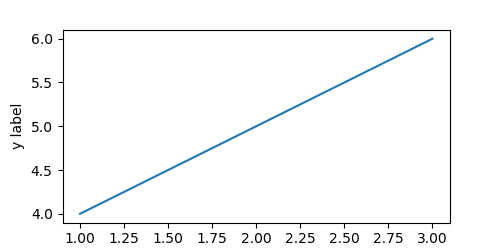It’s the right size in pixels, except that this image is padded weirdly and the x label is cut off (border added).

# Tight Layout

The fix for this is to use a tight layout in the output

plt.figure(figsize=(5, 2.5))
plt.plot([1, 2, 3], [4, 5, 6])
plt.xlabel("x label")
plt.ylabel("y label")
plt.savefig("tight-layout.png", bbox_inches='tight')
print(imread("tight-layout.png").shape) # outputs (259, 462, 4)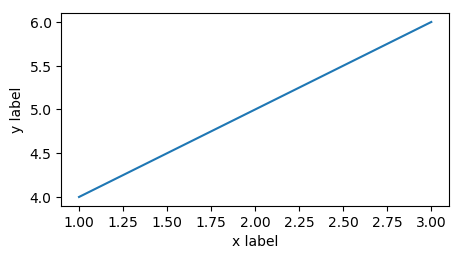This fixes the elements being dropped issue, but the image size is now incorrect (at 2.59x4.62 instead of 2.5x5).

# Fixing the problem

First, we write a general function to get the size of a figure. We then calculate $$x_{\text{to set}} = x_{\text{set previously}} \frac{x_{\text{target}}}{x_{\text{actual}}}$$ for $$x$$ being the width and height. The intuition behind this equation is that we figure out how off the actual image’s size is from our target, and use this to update what we tell matplotlib to do.

However, this is an approximation, and we repeat it to get a better fit. The full code is below:

from matplotlib.image import imread
from tempfile import NamedTemporaryFile

def get_size(fig, dpi=100):
with NamedTemporaryFile(suffix='.png') as f:
fig.savefig(f.name, bbox_inches='tight', dpi=dpi)
return width / dpi, height / dpi

def set_size(fig, size, dpi=100, eps=1e-2, give_up=2, min_size_px=10):
target_width, target_height = size
set_width, set_height = target_width, target_height # reasonable starting point
deltas = [] # how far we have
while True:
fig.set_size_inches([set_width, set_height])
actual_width, actual_height = get_size(fig, dpi=dpi)
set_width *= target_width / actual_width
set_height *= target_height / actual_height
deltas.append(abs(actual_width - target_width) + abs(actual_height - target_height))
if deltas[-1] < eps:
return True
if len(deltas) > give_up and sorted(deltas[-give_up:]) == deltas[-give_up:]:
return False
if set_width * dpi < min_size_px or set_height * dpi < min_size_px:
return False


Using this method (note that we no longer need to set the figure size when creating the figure)

fig = plt.figure()
fig.gca().plot([1, 2, 3], [4, 5, 6])
plt.gca().set_xlabel("x label")
plt.gca().set_ylabel("y label")
set_size(fig, (5, 2.5))
plt.savefig("update-size.png", bbox_inches='tight')
print(imread("update-size.png").shape) # outputs (250, 500, 4)


we get the following result, which is exactly the correct size.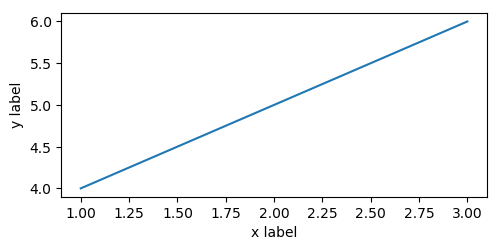# What’s with the return False?

The reason that we check to see if the error is increasing is that sometimes it is impossible to fit a plot within a given space. For example, take the following example

fig = plt.figure()
fig.gca().plot([1, 2, 3], [4, 5, 6])
plt.gca().set_xlabel("x label")
plt.gca().set_ylabel("y label")
set_size(fig, (5, 2.5))
plt.savefig("update-size.png", bbox_inches='tight')
print(imread("update-size.png").shape) # outputs (250, 500, 4)


This ends up trying to decrease the height of the figure to below 0, but before it can cause a crash in matplotlib, it causes the last condition in set_size to be activated, leading to a False return value. The resulting image is 91x109, nowhere close to the 100x50 that it should be, and looks like this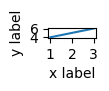By returning False, this allows e.g., a notebook to finish executing in cases where it is unecessary to check, but a simple assert set_size(fig, (1, 0.5)) would lead to an error if that is desired.

# Conclusion

The example above is full, working, code, and should honestly probably be part of matplotlib: generally speaking, when I set the size of a figure, I intend to set the actual size of the figure, not the size of the plot with some minimal padding around it. In any case, hopefully this code is helpful to you!

# Understanding Python's interactive mode

The Python interepreter has this convenient feature that when you type something simple onto the interpreter, it spits that value back out at you, so you can copy/paste it back as a value.

But with convenience often comes complexity, let’s look at how this feature works!

## How does the print function work?

Before we can talk about typing stuff onto the interpreter, let’s talk about the simpler function print. Let’s take a look at a few examples:

>>> print('abcdef')
abcdef
>>> print('1')
1
>>> print(1)
1
>>> x = print(1)
1
>>> x
>>>


So how does print work? Clearly, it returns None once it’s done. But what’s it doing when it’s printing to the screen. Clearly, something strange is happening where both the string '1' and the number 1 get printed out as the single character 1.

To properly understand print, we need to break it down into its constituent parts. Imagine we have a function print_string, which takes a string and prints it to a terminal. Then, we have the following behavior:

>>> print_string('abcdef')
abcdef
>>> print_string('1')
1
>>> print_string(1)
Error


Which is somewhat more consistent. We can implement print_string in terms of print fairly easily as follows:

def print(x):
print_string(str(x))


where str is a function that takes in a value and outputs some string. We have the following behavior for str (illustrated using lists for reasons that will become clear later).

In the case of a string, it does nothing, and in the case of an integer, it converts it into a string. We’ll come back to str later, but for now think of it as providing a nice human-readable version of the given object.

## repr

But if we want an output that isn’t human-readable, but is instead computer-readable, we can use repr instead. For example, repr(1) and repr('1') should produce different outputs as they should be read as two different types. Let’s look at some examples:

>>> print_string(repr('abcdef'))
'abcdef'
>>> print_string(repr('1'))
'1'
>>> print_string(repr(1))
1


Note for later: print_string(repr(<blah>)) prints out <blah>

Looking at an the same example as before using an environment diagram, we have the same result for repr(12) as str(12) but repr('12') has a set of quotes around it while str('12') does not.

This difference can be attributed to the fact that typing in 12 gets us 12, while we need to type in '12' to get '12', whereas 12 appropriately represents both concepts to a human reader.

## Typing something into the interpreter

In the last section, we saw how the repr function works, especially on strings, where it adds a layer of quotes. You might notice that typing something directly onto the interpreter performs a similar task. That’s because it more or less is. We can consider typing something directly into the interpreter (>>> x) to be the same as calling >>> print_string(repr(x)). The examples from above have the same behavior:

>>> 'abcdef'
'abcdef'
>>> '1'
'1'
>>> 1
1


In fact, this is the motivation behind repr, if you type something into the interpreter, it spits it back out at you.

## Typing repr(something) into the interpeter

Let’s say you did the following:

>>> repr('hi')
"'hi'"


What happened? We now have two sets of quotes around the hi. Did the repr function add two sets of quotes instead of one? The answer is no, because, as you can recall from the previous section, that example is equivalent to

>>> print_string(repr(repr('hi')))
"'hi'"


Using our environment diagram, we can see the following:

We see that repr('hi') has four characters in it: ', h, i, and '. repr(repr('hi')) needs to represent this, so it has six characters in it: ", ', h, i, ', ". These six characters get printed to the terminal, to form the string "'hi'".

## __str__ and __repr__

OK, so now that we know that str, repr, print, and typing something directly into the terminal do, let’s look at how these concepts apply to user-defined functions. Take the following class:

class A:
def __repr__(self):
return "hi"
def __str__(self):
return "bye!"


The idea is that whenever we call str(x) when x is of type A, this is equivalent to calling x.__str__(), and whenever we call repr(x) when x is of type A, this is equivalent to calling x.__repr__().

## Examples

Here are some examples of how custom __str__ and __repr__ methods work in practice. Before we get into the explanations, try to figure them out on your own

>>> a = A()
>>> a
hi
>>> print(a)
bye!
>>> repr(a)
'hi'
>>> str(a)
'bye!'


Your first question might be: why no parentheses when we type a into the terminal? The answer is that >>> a is equivalent to print_string(repr(a)), and we have that repr(a) is the string of length 2 containing the letters h, and i; which then gets printed. On the other hand, >>> repr(a) is equivalent to print_string(repr(repr(a))), and repr(repr(a)) is the string of length 4 containing the letters ', h, i, and '. The following environment diagram describes how this process happens:

# Simulationism: Beyond the Matrix

Simulationism is a fairly popular concept. There are many who subscribe to it: from those who use it as a thought experiment to argue against undisprovable theories to those who actually believe we are in a simulation since almost all universes should be simulations. Despite the disparate uses for simulationism, it is generally viewed as a fairly banal concept: the universe is a simulation, and that means absolutely nothing, practically speaking.

However, lurking beneath the seeming simplicity of the idea lies a far more radical set of implications.

## Pure simulationism vs the Matrix

Simulationism is often explained as such: “we’re all living in a simulation, like the Matrix.” For most purposes, this is a good working definition. Mostly, I’ve seen simulationism used as kind of the ultimate Russell’s Teapot: an entirely undisprovable concept. As a Teapot, simulationism is a useful concept because it posits an alternate picture of the world that has no observable differences from ours: this property is true of the Matrix as well (well, apart from the glitches, and the actual plot of the movie).

On the other hand, there’s an important difference between simulationism and the Matrix. In the Matrix, when you wake up, you are you: a real human conciousness produced by a meat brain whose sensory inputs are being manipulated to place you into an alternate reality. However, in simulationism, you can’t wake up, because there is no underlying you: your entire existence, conciousness and sensory experience alike are the interaction between bits of information held in computer memory.

## All is information

This difference is fundamental: simulationism presupposes a philosophical concept that the Matrix does not: all that reality is can be reduced to information. This isn’t quite materialism (in fact, it’s listed as one of the criticisms of materialism on materialism’s Wikipedia article1) but it is similar in its reductionist approach. To many, this seems like a pedantic point: this assumption is an easy one to make (unless you believe in a soul or other such concepts).

## What is a simulation?

So let’s say our universe is indistinguishable from a simulation. What is a simulation? Well, in a conventional sense, a simulation is a program running on data. The program is some set of instructions that allows us to go from one state to another. Take the following very simple world which exists in one of two states: 0 or 1. There are only two possible sets of laws for this universe: the laws that keep it steady or the laws that cause it to flip back and forth. What about 1, 1, 0, 1, 1, 0, ..., you might ask? Well, in that case the first and second 1s are different, which means that there is some additional state that the universe isn’t taking into account.2

For even more complicated universes, we’d want to be able to write down some mathematical formula or algorithm so we could actually run a simulation. It turns out that this isn’t always possible for infinite universes (since the laws might take infinite space to write), but let’s assume the universe is very large but not infinite and thus the rules can be written down.

Now some of you complexity fans out there might be thinking “wait, wait, wait, every set of laws of the universe might be expressible but only some of them would be quickly computable on a concise representation of the universe’s state”. Well, that’s true. But also, does it really matter how slow the simulation runs?3 The concept of time is a product of the laws of the universe: it really shouldn’t matter how long a second inside the universe takes outside the universe.

## Is any running computer a universe?

One of the more interesting things about simulationism is that it allows us to imagine ourselves creating a universe. If we created a powerful enough computer and figured out close enough approximations to the laws and initial state of our own universe, we could set up our own simulation of a universe similar to ours.

But let’s say we didn’t bother with any of that. Let’s say we created a significantly simpler universe than our own on hardware we have sitting on our desk anyway. Is that simulation itself a universe? More generally, any running computer with no input or output from the outside world is a simulation of something, right? It has some state and rules for how to get from one state to another, which was our only definition of a universe.

Is any running computer a universe? Well, we seem to be backed into a corner: the answer has to be yes. One consoling fact is that the universe created by a computer running a screensaver would be nothing like our universe, it would be so dissimilar as to even make such a comparison quite a stretch.

## Do we need the computer?

Let’s say I tell you about a simulation I have in mind: say the state is a number from 0 to 10 (starting at 1) and the law of the universe can be described as the function $f(n) = 2n \mod 11$ You could write a program to simulate this universe: you’d get a cycle of length 10 that went through every state but 0. But that cycle was fixed before you started running the program. Isn’t the mere existence of the program then enough to set up the universe? More concretely, the function $g(n) = 2n \mod 4384165182867240584805930970951575013697$ (credit to UTM for the prime) has more or less the same behavior, though a much longer cycle, which we know even without running the simulation (which would take $$5000$$ times longer than the age of the universe to compute on my desk computer). Does running the simulation even matter if writing it down plans its entire history out anyway?4

So now, a program to calculate the next state, and a first state, written down together comprise a universe.

## Do we need to write it down?

But now we’re in a weird spot: do we need to write the rules of the universe down? I’m not sure what the magic is of writing anything down, really. It’s strange to imagine that a simulation of our universe begins to exist the moment we write it down. The mathematical object that is the universe certainly does not begin to exist: and at this point, what’s the difference between the universe and its representation?

Of course, we’ve gotten to a truly ridiculous point: what this implies is that our universe could be a simulation, not running in some containing universe’s computer, not written down on the page of some containing universe’s book, but simply because it is mathematically possible. Similarly, every other universe with a slight modification of the laws of physics also exists in some other universe. Even more strangely, the universe identical to ours except that on January 1st, 2019, all blue objects turn green exists.5

## If we’re in a simulation, then all things are happening

Simulationism, with a few additional assumptions I’ve made along the way, leads inevitably to a really strong version of multiple universe theory: anything that can be mathematically described exists in some real world. More relevant to our current universe, if every possible set of laws is happening, we don’t know that our universe has parsimonious laws. That means that we are equally likely to be in the “all laws normal” universe as we are to be in the “all blue objects turn green” universe. Thus, allowing for the premise of simulationism implies that scientific induction is literally impossible. The universe looks like it has laws simply by chance, and that fact could change at any minute.

One reason I think a lot of people are relatively comfortable with simulationism is because it seems relatively limited in its implications. In its simplest form, it’s a creator allegory, with the simulation’s programmers taking the place of a more conventional god figure. If thought through to its conclusion, it really is a far more radical idea, with jarring results.

1. For some unfathomable reason under “Quantum mysticism”???

2. The preceeding description, is missing something: what if the simulation isn’t deterministic but is instead random? In that case, we have more possible sets of laws. For example, the next state of the universe could be decided by a coin flip. Or it could be decided by a coin flip with a coin that lands heads only 1/4 of the time, or it could be a combination of the current state and a coin flip. In fact, while there are now an infinite number of laws, we can still characterizing all of them with 4 numbers, that is the probability of staying at 0, staying at 1, moving from 1 to 0, and moving from 0 to 1. The need to have a way to generate randomness, e.g., a coin or a qubit, will be an issue in the future, but for now just assume the computer has access to such a source of randomness.

3. An interesting thought experiment positing vastly different timescales inside and outside the simulation, as well as a nonstandard form of computation, is given by Randall Munroe in a classic xkcd

4. Okay, so I ignored something here, which is the possibility of a nondeterministic program, which produces a different result every time it’s run. However, the only additional assumption we need here is the existence of one standard random string of infinite length (known as the Common reference string model in cryptography). You can think of the common reference string as some fundamental property of the universe, or in practice a deterministic process that’s effectively impossible to predict because it is so complicated. To be honest, this is a fairly large hole in my argument. On the other hand, it seems fairly tenuous to anchor the necessity of a computer within simulationism on the necessity of a universe from which to derive randomness.

5. This would be a possible, though obviously hacky, modification of the laws of our own universe

# Lists and Nonlocal in Python

## Mutable closures

A closure is a function with a parent frame that contains some data. If the parent frame can be modified, the function is said to be mutable.

For example, take the following doctest:

>>> c, d = make_counter(), make_counter()
>>> c()
0
>>> c()
1
>>> c()
2
>>> [c(), d(), d(), d(), c()]
[3, 0, 1, 2, 4]


The c is called multiple times and returns a different value every time; but its state isn’t global as there is the d function which also counts up but on its own tineline.

The Python programming language allows for the creation of mutable closures in two different ways: one that is traditionally considered “functional” and one that is traditionally considered “object-oriented”. Let’s take a look at them now:

## Nonlocal: Functional mutable closures

The most obvious way to implement the make_counter function is as follows:

def make_counter():
current_value = 0
def c():
nonlocal current_value
result = current_value
current_value += 1
return result
return c


The line nonlocal current_value ensures that the current_value variable assigned in the c frame refers to the value from the parent frame. Thus we have shared state (the current_value variable) which can be modified, the necessary components of a closure.

## Lists: Object-oriented mutable closures

But do we really need anything that complicated? What if we did this?

def make_counter():
return [6, 5, 4, 3, 2, 1, 0].pop


Every time we call the resulting function, we get 0, 1, 2, 3, 4, 5, and then 6. This isn’t quite right, as it doesn’t go on forever, but it’s definitely a mutable function, and we never used nonlocal. What’s going on here? Let’s unroll the function somewhat:

def make_counter():
lst = [6, 5, 4, 3, 2, 1, 0]
def c():
return lst.pop()
return c


Here we have that we can modify lst from the parent frame without assigning to it, since the contents of lst rather than the variable itself is mutated. We can exactly duplicate the original make_counter function as such:

def make_counter():
current_value = 
def c():
result = current_value
current_value += 1       # looks like an assignment
return result
return c


Now, this looks like there’s an assignment to current_value on the commented line, but in fact, current_value += 1 is equivalent to current_value = current_value.__iadd__(1), which is equivalent (for numbers) to current_value = current_value + 1, and that is equivalent to current_value.__setitem__(0, current_value + 1), which is not in fact an assignment.

## Complications: Nonlocal on lists

Let’s say we write a few alternatives for make_counter:

def make_counter_append():
current_value = 
def c():
result = current_value[-1]
current_value.append(current_value[-1] + 1)
return result
return c

def make_counter_extend():
current_value = 
def c():
result = current_value[-1]
current_value.extend([current_value[-1] + 1])
return result
return c

def make_counter_plus_equals():
current_value = 
def c():
result = current_value[-1]
current_value += [current_value[-1] + 1]
return result
return c


Interestingly, the first two work but the last does not. Why? Aren’t a += b and a.extend(b) equivalent? Actually, they’re almost equivalent. a += b is equivalent to a = a.__iadd__(b), where __iadd__ is a special function that you can choose to implement on your class. In the case of Python, list.__iadd__ is equivalent to list.extend except that it returns a reference back to the same list.

Therefore, if you want to use a += b where a is a list, you thus need either to make a nonlocal or use a.extend(b) instead.

# Confirming that timezones exist with minimal trust

I was listening to an episode of Oh No, Ross and Carrie! about Flat Earth and it struck me that if you believe the world is conspiring against you, it’s practically quite difficult to prove almost anything. Now one thing that most Flat Earthers do seem to accept is that when its day in one part of the planet, it’s night in another part.

However, let’s say someone denied this. How would you prove it, with the minimum amount of trust?

## Can we do this while trusting nobody?

Let’s say that our experimenter only trusts their own senses. Can they prove that the day/night dichotomy exists? Well, one way they could do this would be to confirm that their watch is correct, then fly to the other side of the world, then confirm that their watch was off by 12 hours from the day/night cycle. However, this isn’t exactly right, it’s possible that someone could mess with your watch to cause it to run slightly fast or slow and cause it to be different when you alived as when you left.

With a fast enough plane, you might be able to confirm that day had turned to night in less than 12 hours, without the need to rely on a possibly manipulable watch. However, the very act of stepping on a plane might allow nefarious agents to manipulate your subjective experience of time, thus fooling you into thinking time zones exist. (Yes, this is a massively unproductive view of the world, but let’s try to work around it.)

Given these limitations, it’s clear that our prover needs a collaborator they can trust.

## Basic protocol with a trusted collaborator

We now have two experimenters, who each trust each other and want to prove the existence of time zones: Alice and Bob. Consider the following protocol: Bob travels across the world while Alice stays behind. Alice and Bob then communicate with each other and confirm that one sees day and the other night.

This works right? Well, almost. The issue is that there’s no protection against a simulation attack: what if Alice speaks to a simulated version of Bob while Bob speaks to a simulated version of Alice, with the two conversations actually taking place 12 hours apart from each other?

Alice and Bob’s position is starting to look undisprovable. But is it?

Let’s say that before leaving, Alice and Bob come up with a shared password, $$p$$, randomly. Then, the protocol is as such:

• Alice and Bob: agree on password $$p$$
• Alice: goes to other side of the world and confirms that its day
• Alice: sends $$p$$ to Bob
• Bob: confirms that its night and that the received message is in fact $$p$$

But wait, the adversary could trap the password after Alice has sent it but before Bob has received it, thus delaying it 12 hours and allowing the protocol to go through, even if the world in fact has no time zones. The issue is that there’s no response from Bob. Bob just sending back the password doesn’t help, since the adversary could do that!

OK, so now we have two different passwords $$p_a$$ and $$p_b$$. The protocol is now as follows:

• Alice and Bob: create passwords $$p_a$$, $$p_b$$ and share them with each other
• Alice: goes to other side of world and confirms that its day
• Alice: sends $$p_a$$ to Bob
• Bob: confirms that its night and that the received message is $$p_a$$
• Bob: sends $$p_b$$ to Alice
• Alice: confirms that it is still the same day and the received message is $$p_a$$

Now, Alice knows for sure that the world in fact has multiple time zones.

However, Alice and Bob might say that the adversary has the ability to read and edit their memories and thus steal their passwords. OK, this objection must make their claim undisprovable, right? In this case the answer is yes, because the adversary could always just convince them that the world had timezones by making arbitrary modifications to their memories.

## Secure Time Synchronization

The issue that Alice and Bob are running into is that they can’t pre-agree on the passwords, because then they know the passwords ahead of time and thus the adversary knows the passwords ahead of time. However, they might be able to “post-agree” on the passwords, as such:

• Alice and Bob: start on opposite sides of the world
• Alice: Confirms that its day
• Alice: Generates and sends $$p_a$$ to Bob
• Bob: Confirms that its night
• Bob: Generates and sends $$p_b$$ to Alice
• Alice: Confirms that it is still the same day
• Alice: meets with Bob
• Alice and Bob: check that all passwords transmitted were correct

If this protocol goes through without Alice and Bob having any issues, then they know that it can be day and night at the same time in different parts of the world. The procedure works!

## Assumptions and Proof

OK, so if we wanted to prove that the above scheme works, we would need a few assumptions. First, we assume Alice and Bob are trustworthy. Second, we need to assume that Alice can confirm that some entity is Bob in a face-to-face conversation and vice versa (so that the Alice and Bob at the end are guaranteed to be the real Alice and Bob). Third, we need to assume that Alice and Bob can tell when night falls, that is, the adversary can’t somehow convince them that two points in time are on the same day when it was in fact night in between those points. Finally, the adversary cannot have time travel and cannot predict either Alice or Bob’s random number generators.

In this case, we can prove that Bob confirming that it is night where he is happened after Alice generated $$p_a$$ and thus during some day D where Alice was, and before Bob generated and thus before Alice received $$p_b$$ and thus before some point on the same day D. Since we assume days are continuous, this proves that Bob observed night during a time when Alice observed day, and thus this procedure is secure.

You can weaken the assumption that two specific trustworthy people are needed by repeating this procedure for every pair of people in some set of N people. Then the scheme proves that time zones exist assuming that 2/N of the people are trustworthy, at the cost of having to repeat the scheme $$O(N^2)$$ times

## Implications

N/A.

Less facetiously, I guess my general takeaway is that “your claim is undisprovable” shouldn’t be used as a synonym for “you’re making ridiculous, conspiratorial, assumptions”. Because even when someone is being paranoid, there might be a way to disprove their hypothesis, with enough effort.

# Infix Notation in Scheme

Scheme is well known as a very simple programming language. The general concept behind scheme is that it is composed of S-expressions, or expressions of the form (operator operand1 operand2 ... operandn), which can be nested.

This makes scheme relatively simple to parse and evaluate, however it does lead to some strange compromises on the style of programming. For example, rather than writing 12 * (2 + 3), you have to write (* 12 (+ 2 3)). And in a way, this is elegant in its simplicity.

But simplicity is overrated.

## Homoiconicity, or writing a scheme program to write a scheme program

Scheme has this cool property of being a homoiconic language. This means that you can write scheme code that writes and modifies scheme code.

For example, take this function, which replaces the first element form a scheme expression with a +:

(define (replace-with-plus code)
(cons '+ (cdr code)))


Note that we just treat the code like any other scheme list. To call this function, we can just run

scm> (replace-with-plus '(- 2 3))
(+ 2 3)


Now, if we want to actually run this program, we can wrap it in an eval call as such:

scm> (eval (replace-with-plus '(- 2 3)))
5


## Macros

You’ll note that in the above, we have to quote the arguments and then eval the result. It turns out that there’s a construct in Scheme that will do this for you! Using the same replacing with + function, we can define

(define-macro (replace-with-plus code)
(cons '+ (cdr code)))


Note that this is the same definition, except that we have replaced define with define-macro. Now, Scheme handles the quoting of the arguments and the evaluation of the result for us as such:

(replace-with-plus (- 2 3))
5


Pretty nifty, huh!

## Infix notation

OK, but the post’s title is infix notation. Let’s say we want to be able to write (12 * (2 + 3)) in scheme and have it evaluate the way it would in Python. To accomplish this, let’s define a function (not a macro for now) that takes in (12 * (2 + 3)) and converts it into the scheme form (* 12 (+ 2 3)). First off, we need to identify which things are in fact infix notation:

(define (infix? code)
(and
(list? code)
(= (length code) 3)
(member (cadr code) '(+ * - /, >, <, >=, <=, =))))


The idea here is that we check that the given piece of code is a list with 3 elements, and that its middle element is an operator. Now, let’s write the code to convert infix to prefix notation.

(define (rearrange code)


Where this code just places the elements in second, first, third order.

Now, we can put it together with a recursive function:

(define (to-prefix-func code)
(cond
((infix? code) (to-prefix-func (rearrange code)))
((list? code) (map to-prefix-func code))
(else code)))


The idea behind this code is that if something is infix, we should rearrange it, then call ourselves to finish up the task, if something is a call expression, we should just look at every element, and otherwise, we’re at a base case and can just return.

Let’s try this out on our example:

scm> (to-prefix-func '(12 * (2 + 3)))
(* 12 (+ 2 3))


It works!

Finally, what we really want is a macro. We can just define one directly:

(define-macro (to-prefix code) (to-prefix-func code))


Sadly we can’t just (define-macro to-prefix to-prefix-func) because of a technicality in the scheme language. Anyway, now we can run our scheme code:

scm> (to-prefix (12 * (2 + 3)))
60


And there you have it. Scheme’s power might be in its simplicity but with great power comes the ability to create complexity. Obviously, the usual disclaimer applies: don’t write code like this, but if you do, do it with macros!

## The code

A fully runnable version of this is below: try copy-pasting it into 61A’s scheme interpreter and check it out! (There are a few helpful functions defined at the end that we just kinda assumed the existence of above).

(define (infix? code)
(and
(list? code)
(= (length code) 3)
(member (cadr code) '(+ * - /, >, <, >=, <=, =))))

(define (rearrange code)

(define (to-prefix-func code)
(cond
((infix? code) (to-prefix-func (rearrange code)))
((list? code) (map to-prefix-func code))
(else code)))

(define-macro (to-prefix code)
(to-prefix-func code))

(define (member elem lst)
(cond
((null? lst) #f)
((eq? (car lst) elem) #t)
(else (member elem (cdr lst)))))

(define (cadr lst) (car (cdr lst)))
(define (cddr lst) (cdr (cdr lst)))
(define (caddr lst) (car (cddr lst)))


# (Mini) Scheme in 50 Lines

The scheme language is a programming language that is unique for being easy to parse. Every construct in scheme is of the form

(keyword arg1 ... argn)


## Our Subset

We will start with some standard scheme expressions, which you can think about as being analogous to certain patterns in Python. For example instead of writing a + b, you write (+ a b), where + is just the name of a function. Similarly, instead of writing lambda x: x * 2 for the doubling function, you write (lambda (x) (* 2 x)). Just lambdas and function application are enough to perform any calculation, but we add a few more features for clarity: instead of x = y we write (define x y), instead of x if y else z we write (if y x z) and instead of writing

x = f(y)
return x


we write (begin (define x (f y)) x). The real scheme language has many more constructs, including ones that simulate python’s def statements, and some unique ones that allow you to assign variables in a local frame, simulate elif trees, have short-circuited and/or constructs, or even define your own language constructs. For brevity, we will stick to this subset, which is still very powerful.

## Lexing

The first step in processing a scheme program is to split it up into a list of tokens. What we do is take an expression like '(a b (c))' and convert it into a list of tokens like '(', 'a', 'b', '(', 'c', ')', ')'.

To accomplish this, we first pad all the parentheses with space and replace all space-like characters with a space. We then split on spaces (and filter out empty tokens, which are produced when multiple spaces are consecutive).

def lex(text):
text = re.sub("([()])", r" \1 ", re.sub(r"\s", " ", text))
return [x for x in text.split(" ") if x]


## Parsing

We now need to parse the string. If we have '(a b (c))', we want to parse it to ['a', 'b', ['c']] so we can process it in Python. Parsing scheme is fairly simple using recursion. Whenever we see a symbol or number, we just return and move on. Otherwise, we recursively call parse until we see an end parenthesis. For example, if we have the current state of our input

(+ 2 (4) (* 5 3)) 2 3)


we parse by removing the ( from the front, then parse +, 2, (4), and (* 5 3) before seeing the unmatched ). Then we return, having removed everything but the 2 3). This is implemented as follows:

def parse(text):
text = lex(text)[::-1]
def parse():
start = text.pop()
if start != '(':
return start
vals = []
while text[-1] != ')':
vals += [parse()]
text.pop()
return vals
return parse()


The reason we reverse the text is so that pop() pops from the end rather than the front of the input stream.

## Frames

We need to define an environment diagram so that we can execute code. This is similar to a Python style environment diagram, which is a backwards pointing tree (children point towards the root via the parent annotation rather than the other way around).

To define a frame, we use the defaultdict class from python, which is like a dictionary except that when it can’t find a key, instead of raising a KeyError, it calls a 0 argument function you provide in the constructor and sets that as its value. We want to slightly modify this so that it calls a 1 argument function. Thus we define a frame as such:

class frame(defaultdict):
def __init__(self, f):
super().__init__(lambda: None)
self.__function = f
def __missing__(self, key):
return self.__function(key)


defaultdict calls the __missing__ function when necessary, and in this case, it calls the parent function on a key. We can create a child frame for a given frame simply by saying frame(lambda v: parent[v]). We can now create a global frame as such:

global_frame = frame(int)
global_frame.update({"+" : add, "-" : sub, "*" : mul, "/" : floordiv, "=" : lambda x, y: x == y})


We do something a little hacky here by basically defining integers as just being variables that evaluate to the integer version of themselves via the function int. (this works as int("123") == 123).

## Special Forms

Now we need to somehow handle our special keywords (define, lambda, begin, if).

### Define

We assume that we already have a function seval defined, which is the function that will eval a scheme expression. In scheme, define returns the variable being assigned to, for reasons of tradition.

def define(exp, env):
env[exp] = seval(exp)
return exp


### Lambda

A lambda expression has no side effects, but must return a function. First we create a new frame, then we assign the operator to the operands, and then we run the body of the function in that frame and return the last value:

def slambda(exp, env):
def do_lambda(*args):
local_env = frame(lambda x: env[x])
local_env.update(dict(zip(exp, args)))
return [seval(u, local_env) for u in exp[1:]][-1]
return do_lambda


### Begin

This is similar to lambda except that we don’t have any arguments or a new frame, and this one is simple enough to just write as a Python lambda function.

lambda exp, env: [seval(u, env) for u in exp][-1]


### If

We can just directly map this to the equivalent Python construct.

lambda exp, env: seval(exp, env) if seval(exp, env) else seval(exp, env)


### Putting it together

We create a dictionary of special forms for easy access:

def define(exp, env):
env[exp] = seval(exp)
return exp
def slambda(exp, env):
def do_lambda(*args):
local_env = frame(lambda x: env[x])
local_env.update(dict(zip(exp, args)))
return [seval(u, local_env) for u in exp[1:]][-1]
return do_lambda
special_forms = {
"define" : define,
"lambda" : slambda,
"begin" : lambda exp, env: [seval(u, env) for u in exp][-1],
"if" : lambda exp, env: seval(exp, env) if seval(exp, env) else seval(exp, env)
}


## Eval Function

To evaluate a parsed scheme tree, what we need to do is dependent on whether the input is a list or not. If we have a list, then we need to check if its first element is a special form, and if so run its special form function. Otherwise, we evaluate the first item as a function, then evaluate the rest of the items as its arguments, then call the function. If we don’t have a list, we just look up the current element in the current frame.

def seval(tree, env=global_frame):
if isinstance(tree, list):
func, *args = tree
if func in special_forms:
return special_forms[func](args, env)
return seval(func, env)(*(seval(x, env) for x in args))
return env[tree]


We can then run scheme by running seval(parse(text)).

## The Entire Interpreter

The entire interpreter, which is just 50 lines, is as below:

from collections import defaultdict
from operator import *
import re

def lex(text):
text = re.sub("([()])", r" \1 ", re.sub(r"\s", " ", text))
return [x for x in text.split(" ") if x]
def parse(text):
text = lex(text)[::-1]
def parse():
start = text.pop()
if start != '(':
return start
vals = []
while text[-1] != ')':
vals += [parse()]
text.pop()
return vals
return parse()
class frame(defaultdict):
def __init__(self, f):
super().__init__(lambda: None)
self.__function = f
def __missing__(self, key):
return self.__function(key)
global_frame = frame(int)
global_frame.update({"+" : add, "-" : sub, "*" : mul, "/" : floordiv, "=" : lambda x, y: x == y})

def define(exp, env):
env[exp] = seval(exp)
return exp
def slambda(exp, env):
def do_lambda(*args):
local_env = frame(lambda x: env[x])
local_env.update(dict(zip(exp, args)))
return [seval(u, local_env) for u in exp[1:]][-1]
return do_lambda
special_forms = {
"define" : define,
"lambda" : slambda,
"begin" : lambda exp, env: [seval(u, env) for u in exp][-1],
"if" : lambda exp, env: seval(exp, env) if seval(exp, env) else seval(exp, env)
}
def seval(tree, env=global_frame):
if isinstance(tree, list):
func, *args = tree
if func in special_forms:
return special_forms[func](args, env)
return seval(func, env)(*(seval(x, env) for x in args))
return env[tree]


Of course, a proper scheme interpreter, which has more features (see the section “Subset”), more possible types (like strings, symbols, lists, etc.), and better error handling (we crash on a lot of cases with IndexErrors and ValueErrors). In fact, that’s an entire project in 61a.

But I personally enjoy that you can get quite a few features in such a small amount of space.

Try it out on the following program!

(begin
(define factorial
(lambda (x) (if (= 0 x) 1 (* x (factorial (- x 1))))))
(factorial 40))


# The Efficiency Gap Makes No Sense (but all metrics show Republicans are better at gerrymandering)

## Cracking, Packing, and Wasted Votes

The efficiency gap is a technique for measuring the level of gerrymandering in a state. Gerrymandering generally takes the form of what’s known as “cracking and packing.” Cracking is the process of taking a district with a majority of the opposing party and splitting it into two in which the party has a minority in each, thus denying your opponent that seat. However, this isn’t always possible because your opponent might have too many votes in a given area. To reduce the remaining number of votes your opponent has while minimizing the number of seats they have, you can use a technique called packing, in which you create a district in which your opponent can win by a huge margin.

To measure both cracking and packing, we can use the concept of a wasted vote. A wasted vote is any vote that did not contribute to the victory. There are two kinds of wasted votes, cracked waste and packed waste. A cracked wasted vote for party X is any vote in a district party X has lost. A packed wasted vote is any vote for party X over 50% in a district party X has won.

For example, let’s say that there are just two parties1 and 1000 voters in a district. The number of wasted votes for X in terms of the number of votes for X is as such:1. The United States has two political parties. A vote for the Green, Libertarian, or American Independent Party is a wasted vote. But if you’re voting for the AIP (the party that nominated Wallace), you should waste your vote, so I guess I’m fine if you vote for the AIP.

# How You Get Homeopathy

Homeopathy is a system that has two primary claims. The first is the law of similars. It says that a dilute solution of a substance cures the ailment caused by a concentrated solution of the same substance. The second is the law of dilution, which says that the more dilute a homeopathic preparation is, the stronger its effect.

The second theory is the one people tend to focus on because it is clearly incorrect. The anti-homeopathy $$10^{23}$$ campaign is named after the order of magnitude of Avogadro’s number, that is the number of molecules of a substance in a 1M solution of a substance (typically a fairly strong solution, 1M saltwater is 5 teaspoons of salt in a liter of water). If you dilute by more than that amount, and many “strong” homeopathic preparations do, then with high probability you literally have 0 molecules of the active ingredient in your preparation. As the $$10^{23}$$ campaign’s slogan makes clear, “There’s nothing in it” and homeopathic medicines are just sugar pills.

# What is NP?

## What is P?

This seems like a silly question. This question seems easily answered by the surprisingly comprehensible explanation on P’s Wikipedia page:

It contains all decision problems that can be solved by a deterministic Turing machine using a polynomial amount of computation time

OK, so it’s the set of all decision problems (yes-or-no questions) that are $$O(n^k)$$ for some integer $$k$$. For example, sorting, which is $$\Theta(n \log n)$$ is P, since it’s also $$O(n^2)$$.

But let’s go for an alternate definition. Let’s take a C-like language and get rid of a bunch of features: subroutine calls, while loops, goto, for loops with anything other than i < n in the second field (where n is the length of the input), and modifying the for loop variable within the loop. Effectively, this language’s only loping construct is for(int varname = 0; varname < n; varname++) {/* Code that never modifies varname */}. Let’s call this language C-FOR.

# Storing Data With the Circle-Tree

## The Pair Abstraction

Computers need some way to store information. More specifically, we want to be able to store multiple pieces of data in one place so that we can retrieve it later. For now, we’ll look at the simplest way of storing data, a pair.

To express the concept of a “pair”, we need three machines.

1. A way to put two things together (construction, or “C” for short)
2. A way to get the first element (first, or “F” for short)
3. A way to get the second element (second, or “S” for short)

# Using Circle-Tree Drawings as a Calculator

So this isn’t just a procrastination game for doodling when you’re bored (and have a lot of colored pencils, for some reason). We can use circle-tree as a way to express math!

## Numbers

For math, the first thing we need are numbers. I’m just going to draw some pictures which are defined to be the numbers. We don’t worry too much about whether they are “really” numbers or not in the way that we don’t worry about whether “102” or “CII” is a better way to explain the concept of “how many cents in a dollar plus how many hands a person has”ness.1

So this is 0:1. Although, off the record, for doing math CII is a terrible way of representing 102.

# Introducing the Circle-Tree System

If you want to follow along on paper, you’ll want some colored pencils. Like at least 5 colors, not including a pencil to draw links.

# Circtrees

In any case, we’ll call the diagrams we’re interested in “circtrees.”1 Circtrees can be constructed in one of three ways.

## 1. Dots

A dot of any color is a circtree. Here are some examples2:1. You can probably guess where that name came from

2. I choose to draw pretty big dots, but if you’re doing this by hand, you’ll probably want to use smaller ones for ease of drawing.

# Counting Monoids

## What’s a Monoid?

A monoid is a set $$M$$ with an identity element $$e : M$$ and an operation $$* : M \to M \to M$$, where we have the laws:

• $$x * e = x$$
• $$e * x = x$$
• $$x * (y * z) = (x * y) * z$$

We write a monoid as a tuple $$(M, e, * )$$. Some example monoids (written in pseudo-Haskell) are

(Int, 0, (+))
(Int, 1, (*))
(Bool, True, (||))
((), (), \x y -> ())
([a], [], (++))


# The Porus Mirror Problem, Part 2

## Propagation

From last time, we know the quantity of light that escaped at each location and direction around the unit circle. We want to find how this translates into quantities of light at any point away from the circle. This situation can be modeled as so:# The Porus Mirror Problem, Part 1

## The porus mirror problem

I’m pretty sure I came up with this problem on my own, but I’m not sure if its an existing problem under a different name. Anyway, the situation is as such:

1. We have a very short cylindrical tube. In fact, we’ll ignore the third dimension and just use a circle. For simplicity, we’re using units such that the radius is 1.
2. The tube is filled with a gas that emits corpuscles in one large burst. We assume a large enough number that we can model them continuously. We additionally assume that the emmissions are uniform in location and direction.
3. The tube itself is semitransparent, with transparency determined by a function $$t: [0, 2\pi] \to [0, 1]$$ of angle around the circle. Any light that does not pass through is reflected across the normal.

We wish to calculate at each point along the surface of the tube what is the total quantity of light emitted in each direction, from which we can easily calculate the light pattern this system will cast on any shape outside.

# Dependent Types and Categories, Part 1

So I’ve been trying to go through Category Theory for Scientists by writing the functions as code. So I obviously decided to use Haskell. Unfortunately, I ran into a wall: Haskell is actually pretty bad at representing categories.

Now, at first this was incredibly surprising. Frankly, I had started learning about category theory because of its association with Haskell. Unfortunately, I didn’t realize that Haskell isn’t category theory, it’s a category.

For instance, look at this definition of a category in math:

# Chaos, Distributions, and a Simple Function

$$\newcommand{\powser}{\left{ #1 \right}}$$

## Iterated functions

The iteration of a function $$f^n$$ is defined as:

$f^0 = id$ $f^n = f \circ f^{n-1}$

For example, $$(x \mapsto 2x)^n = x \mapsto 2^n x$$.

Most functions aren’t all that interesting when iterated. $$x^2$$ goes to $$0$$, $$1$$ or $$\infty$$ depending on starting point, $$\sin$$ goes to 0, $$\cos$$ goes to something close to 0.739. $$\frac{1}{x}$$ has no well defined iteration, as $$(x \to x^{-1})^n = x \to x^{(-1)^n}$$ which does not converge.

OK, so the real reason I covered Products and Coproducts last time last time was to work toward a discussion of limits, which are a concept I have never fully understood in Category Theory.

## Diagram

As before, we have to define a few preliminaries before we can define a limit. The first thing we can define is a diagram, or a functor from another category to the current one. In Haskell, we can’t escape the current category of Hask, so we instead define a diagram as a series of types and associated functions. For example, we can define a diagram from the category $$\mathbf 2$$ containing two elements and no morphisms apart from the identities as a pair of undetermined types.

A diagram from the category $$\mathbf 2*$$, a category containing two elements and an arrow in each direction (along with the identities), (this implies that the two arrows compose in both directions to the identitity):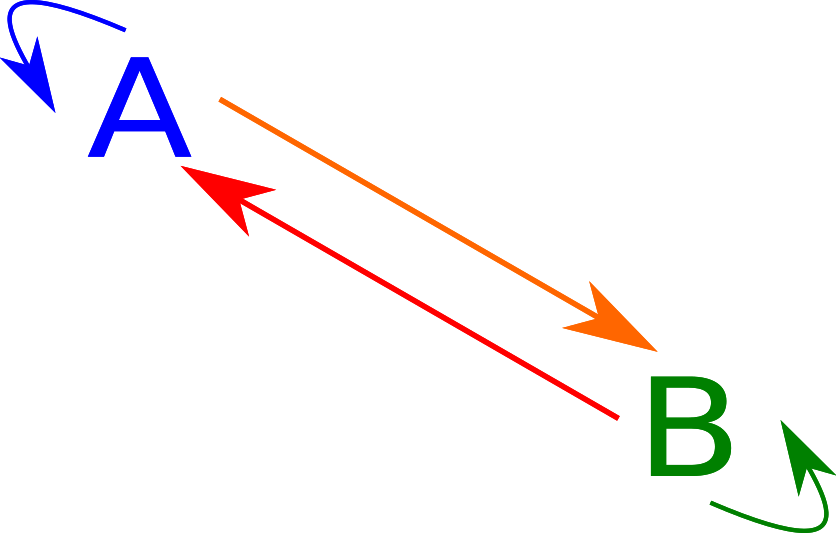# Haskell Classes for Products and Coproducts

## Product Data Structures

Product Types are a familiar occurrence for a programmer. For example, in Java, the type int $$\times$$ int can be represented as:

class IntPair {
public final int first;
public final int second;
public IntPair(int first, int second) {
this.first = first;
this.second = second;
}
}


# Monoids, Bioids, and Beyond

## Two View of Monoids.

### Monoids

Monoids are defined in Haskell as follows:

class Monoid a where
m_id :: a
(++) :: a -> a -> a


Monoids define some operation with identity (here called m_id). We can define the required laws, identity, and associativity, as follows:

monoidLaw1, monoidLaw2 :: (Monoid a, Eq a) => a -> Bool
monoidLaw1 x = x ++ m_id == x
monoidLaw2 x = m_id ++ x == x

monoidLaw3 :: (Monoid a, Eq a) => a -> a -> a -> Bool
monoidLaw3 x y z = (x ++ y) ++ z == x ++ (y ++ z)


# Nontrivial Elements of Trivial Types

We know that types can be used to encode how to make useful data structures.

For example, a vehicle can be encoded as:

data Vehicle = Car { vin :: Integer, licensePlate :: String }
| Bike


In this case, valid values include cars with VINs and LPNs, or Bikes, which have neither of these data points.

However, some data types can seem far less useful.

# The Autogeneration of Landscapes

OK, so a break from theory and CS, and to applications. How do we produce reasonably realistic landscapes using differential equations?

## Mountains and Valleys

To simulate mountains and valleys, we can use a fairly simple algorithm that adds a random amount to a region, then recursively calls itself on each quadrant of the region. Afterwards, an image generally looks something like: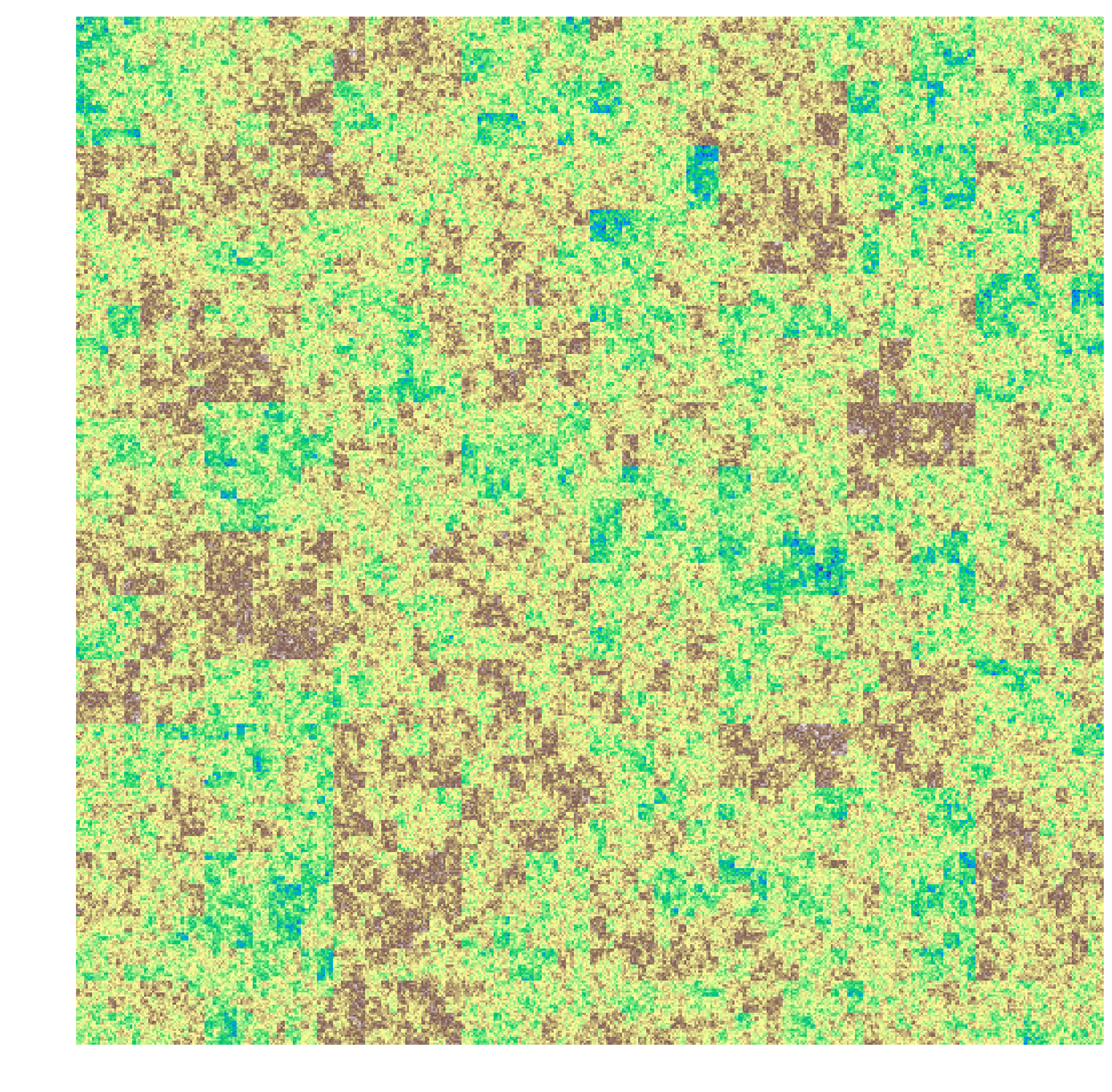We can apply a Gaussian blur to the image to get a reasonably realistic depiction of mountains and valleys.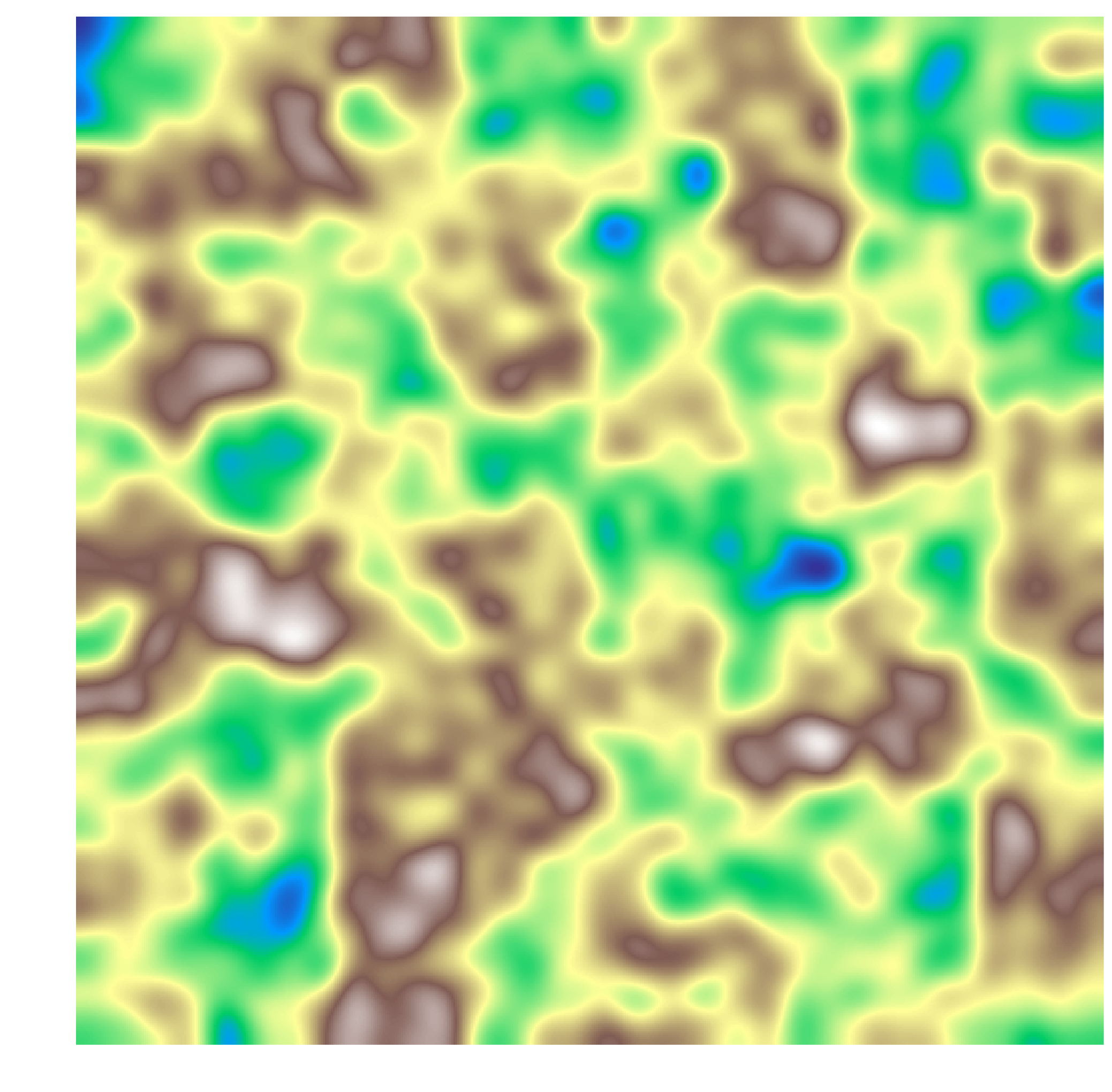We can define the elevation as a function $$E(x, y, t)$$. Representing this as a landscape: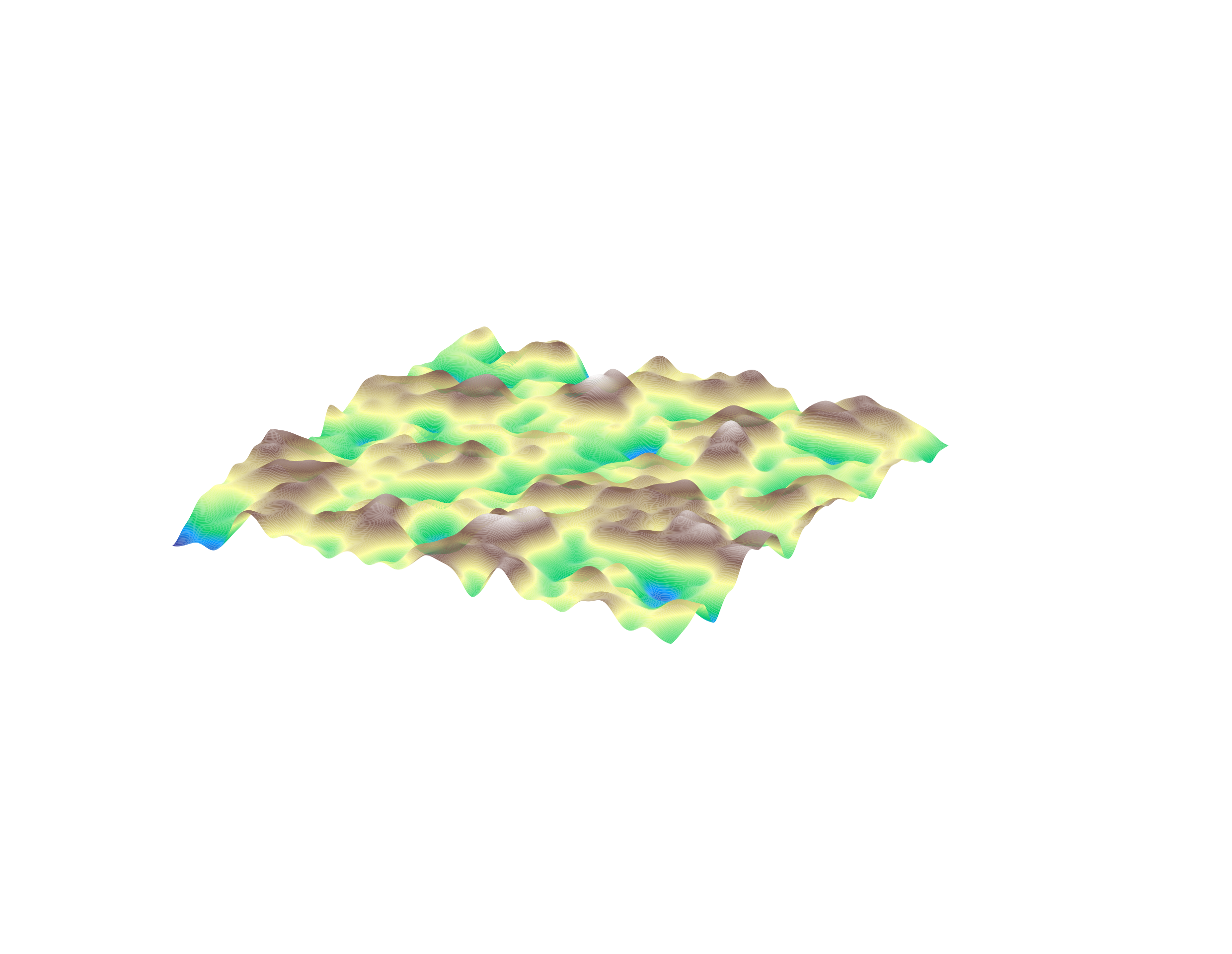# C-Style Pointer Syntax Considered Harmful

## Declaration Follows Use

In C, a pointer to int has type int*, but really, this isn’t the right way of looking at it. For example, the following declaration:

int* x, y;


creates x of type int* and y of type int.

# The Triviality Norm

Feynman famously said that to a mathematician, anything that was proven was trivial. This statement, while clearly a lighthearted dig at mathematics, it does have a striking ring of truth to it.

## Logical Implication

In logic, there is the concept of implication. The idea is that if $$B$$ is true whenever $$A$$ is true we can say that $$A \implies B$$. This makes sense for statements that depend on a variable. For example, we can say that $$x > 2 \implies x > 0$$.

# A Monadic Model for Set Theory, Part 2

$$\newcommand{\fcd}{\leadsto}$$

Last time, we discussed funcads, which are a generalization of functions that can have multiple or no values for each element in their input.

The composition of funcads is defined to be compatible with the composition of functions, and it takes a fairly similar form:

$f :: Y \fcd Z \wedge f :: X \fcd Y \implies \exists (f \odot g :: X \fcd Z), (f \odot g)(x) = \bigcup_{\forall y \in g(x)} f(y)$

In other words, map the second function over the result of the first and take the union. If you’re a Haskell programmer, you probably were expecting this from the title and the name “funcad”: the funcad composition rule is just Haskell’s >=>.

# A Monadic Model for Set Theory, Part 1

$$\newcommand{\fcd}{\leadsto}$$

## Solving an Equation

A lot of elementary algebra involves solving equations. Generally, mathematicians seek solutions to equations of the form

$f(x) = b$

The solution that most mathematicians come up with to this problem is to define left and right inverses: The left inverse of a function $$f$$ is a function $$g$$ such that $$g \circ f = \mathrm {id}$$. This inverse applies to any function that is one-to-one. There is also a right inverse, a function $$g$$ such that $$f \circ g = \mathrm {id}$$ For example, the left inverse of the function

$x \mapsto \sqrt x :: \mathbb R^+ \rightarrow \mathbb R$

is the function

$x \mapsto x^2 :: \mathbb R \rightarrow \mathbb R^+$

# What is Category Theory?

$$\newcommand{\mr}{\mathrm}$$ So, I was reading Category Theory for Scientists recently and kept having people ask me: What is category theory? Well, until I hit chapter 3-4, I didn’t really have an answer. But now I do, and I realize that to just understand what it is doesn’t really require understanding how it works.

## Sets and functions

So, set theory is all about sets and functions. Why are we talking about set theory and not category theory, you may ask? I’ll get to category theory eventually, but for now, let’s discuss something a little more concrete.

Let’s look at subsets of the set $$\{a, b\}$$. We have the possibilities, which we name Empty, A set, B set, Universal set:

$E = \{\}, A = \{a\}, B = \{b\}, U = \{a, b\}$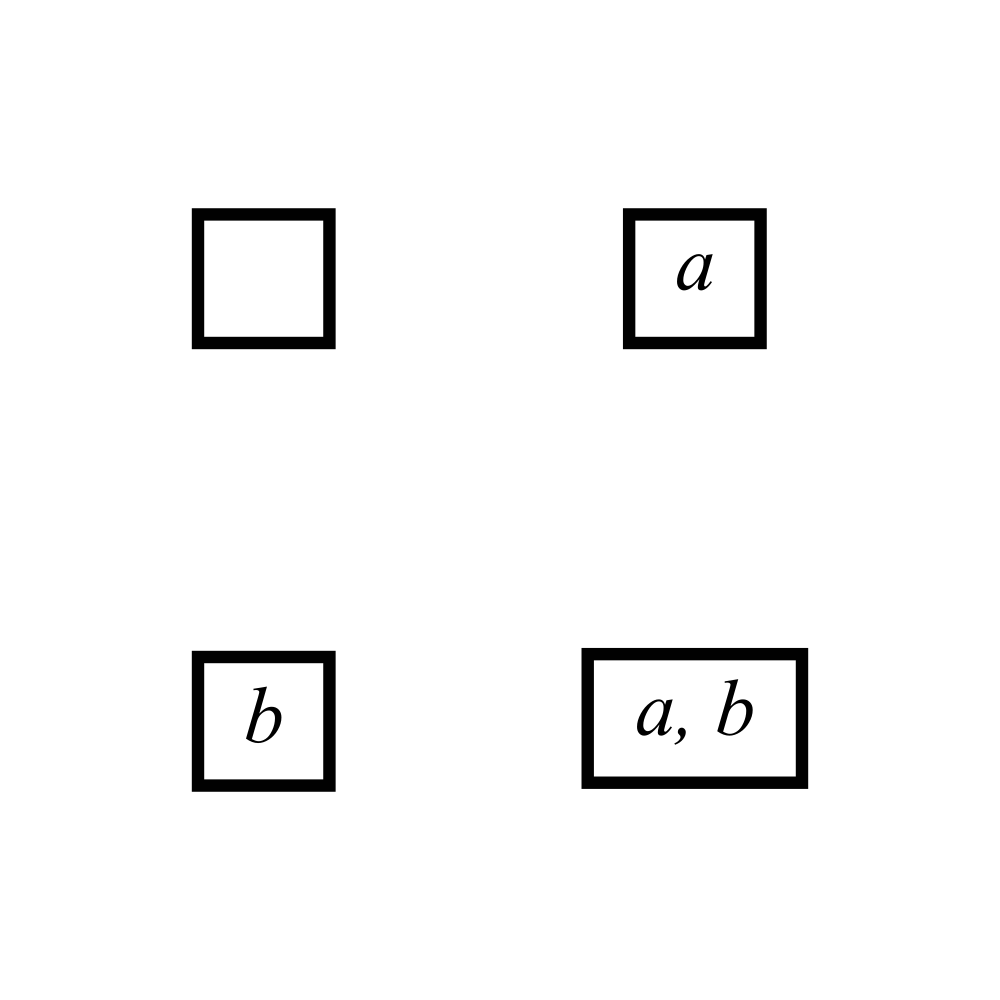# Stupid Regex Tricks

## Regexes, the duct tape of CS

Regexes are truly amazing. If you haven’t heard of them, they are a quick way to find (and sometimes replace) some body of text with another body of text. The syntax, while it has its critics, is generally pretty intuitive; for example a zip code might be represented as

[0-9]{5}(\s*-\s*[0-9]{4})?


which is read as “a five digit code optionally followed by a spaced dash and a four digit code”. Some whitespace and named groups might help the reading (use COMMENTS mode when parsing)

(?P<main_code>
[0-9]{5}
)
( # optional extension
(?P<optional_extension>
[0-9]{4}
)
)?


# Dictp Part 2

OK, so to continue the Dictp saga, some syntactic sugar.

## References to local variables.

The parser can be enhanced by rewriting anything that isn’t a built-in command or a string with a lookup to __local__.

E.g.,

• a $$\mapsto$$ __local__['a']
• 0 = 1 $$\mapsto$$ __local__['0'] = __local__['1']
• 0? $$\mapsto$$ __local__['0']?
• '0' $$\mapsto$$ '0'

# Dictp Part 1

So, a friend and I (I’m going to protect his anonymity in case he doesn’t want to be associated with the travesty that is what we ended up thinking up) were discussing ridiculous ideas for programming languages: you know the deal, writing C interpreters in Python, using only integers to represent everything, etc.

One idea, however, seemed a little less ridiculous to us (OK, just to me): a language in which there are two data types: Dictionary and String.

# Infsabot Strategy Part 2

OK, so to continue our Infsaboting.

### Correction

I made a mistake last time. I included SwitchInt, a way to switch on values of ExprDir, which is ridiculous since an ExprDir can only be constructed as a constant or an if branch to begin with.

So just imagine that I never did that.

## Guess and Check

OK, so how should our AI construct these syntax trees? At a very high level, we want to be able to 1) assess trees against collections of trees and 2) modify them randomly. We can represent this as a pair of types:

asses :: [RP] -> RP -> Double
modify :: RP -> StdGen -> (RP, StdGen)


I think the implementation of asses should be pretty clear: simply find the win rate (given that we can simulate an unlimited number of games).

Modify, on the other hand, is a little more complicated. There are a few ways a tree can be modified:

• Modify the value of a constant to be something else.
• Modify the value of a field to point to something else or a field
• Add leaves to the tree
• Remove leaves as a simplification step

# Infsabot Strategy Part 1

OK, so what is this Infsabot? Infsabot is a game I designed for people to write programs to play. Basically, it’s like chess, except where each piece acts independently of each other.

## The Game

There is a square board where each square is either empty or contains material. The game pieces, robots, require material to stay alive and perform tasks. At each turn, every robot reports which of the following it wants to do:

• Noop: The robot does nothing
• Die: The robot dies
• Dig: The robot digs to try to extract material
• Move: The robot can move in one of the four compass directions
• Fire: The robot can fire a quick-moving projectile (e.g., travel time = 0) in a compass direction
• Message: The robot can send a quick-moving message in a compass direction
• Spawn: The robot can create a new robot with its own initial material and appearance

To decide what to do, a robot has access to two things:

• A picture of the positions around it, to a given distance
• Whether or not the spot contains material
• Whether or not the spot contains a robot, and it’s appearance (not it’s team)
• A snapshot of its own hard drive, which is a massive relational database between Strings and Strings
• A list of all messages received

# What's a number?

OK, so a bit of a silly question. Of course, we all know what numbers are. But humor me, and try to define a number without using “number”, “quantity”, or anything that refers back to a number itself.

But, before we learn numbers, we have to first understand something simpler: counting. Couldn’t we define numbers in terms of counting? Well, yes, otherwise I wouldn’t be writing this article.

Now, to not end up with a forty page book, I’m only going to go over how to define the whole numbers (I’m going to ignore negative integers, fractions, $$\pi$$, etc.)

# Recursivized Types

So, a short break from $$\varepsilon—\delta$$.

Anyway, I’m going to look at Algebraic types in this section. Specifically, I am going to use Haskell types.

The provided code is all actual Haskell, in fact, I’m going to have to de-import the standard libraries because some of this is actually defined there. You can find all the code here

# Who Needs Delta? Defining the Hyper-Real Numbers

$$\renewcommand{\ep}{\varepsilon}$$ $$\renewcommand{\d}{\mathrm d}$$

In high school Calculus, solving differential equations often involves something like the following:

$\frac{\d y}{\d x} = \frac{y}{x}$

$\frac{\d y}{y} = \frac{\d x}{x}$

$\int \frac{\d y}{y} = \int \frac{\d x}{x}$

$\log y = c + \log x$

$y = kx$

Now, I don’t know about anyone else, but this abuse of notation really bothered me at first. Everyone knows that $$\d x$$ isn’t an actual number by itself, but we seem to use it all the time as an independent variable.

# Ancient Greek Abstract Algebra: Introduction

Note: I’ve put the Ancient Greek Abstract Algebra series on hold for now. Please take a look at some of my other post(s).

## Modern constructions of $$\mathbb{N}$$

The natural numbers are taught to children as a basic facet of mathematics. One of the first things we learn is how to count. In fact, the name “Natural Numbers” itself implies that the natural numbers are basic.

However, modern mathematicians seem to have trouble with accepting the natural numbers as first class features of mathematics. In fact, there are not one but two common definitions of the naturals that can be used to prove the Peano Axioms (the basic axioms from which most of number theory can be proven).